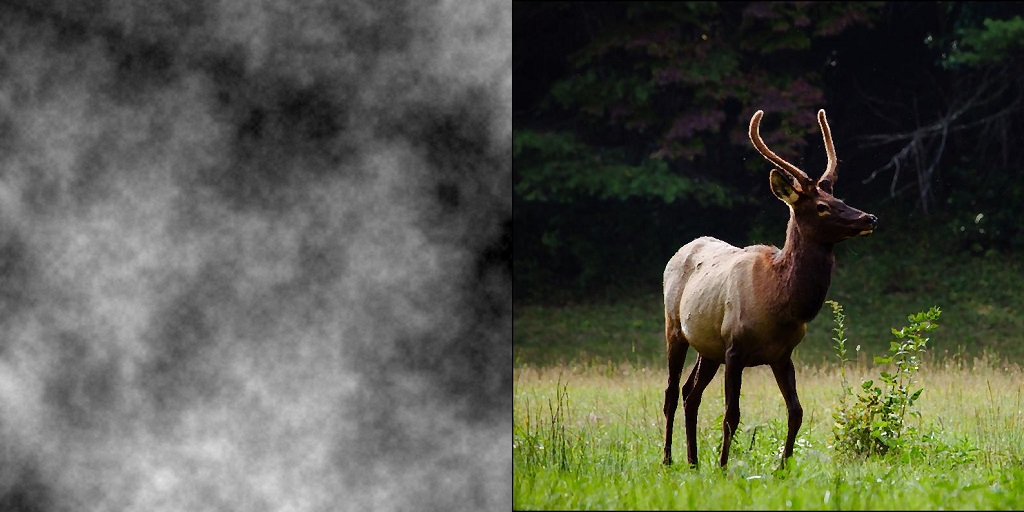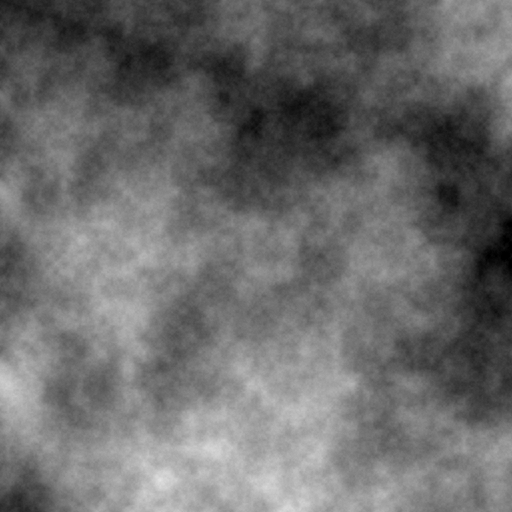### Image processing on a GPU with AForgeAForge is a popular .Net library for image processing and computer vision. It features a lot of image filters, noise generators, or other image-related algorithms.
Since it’s written in C#, and given the very parallel nature of most of image processing algorithms, we tried to hybridize some of them.
AForge code make heavy use of dot net `unsafe`, which is supported by the Hybridizer.

We chose two examples to illustrate hybridizer’s capabilities. First is Perlin noise, a highly compute intensive noise generator, and second is AdaptiveSmoothing, a latency bound image filter.

## Perlin noise

Perlin noise is written for double precison in AForge, and we test on a GTX 1080 Ti, which has almost no double precision arithmetic units. We therefore first cloned the Perlin noise algorithm and made a PerlinNoiseFloat class, in which we just replaced “double” by “float”, without changing a single bit of the code structure or algorithm implementation.
However, Perlin noise makes use of Cosine function, which is only available for double in C#. We replaced Math.Cos by an Intrinsic function, mapped to cosf from C99 standard:

```[IntrinsicFunction("cosf")]
public static float cosf(float x)
{
return (float)Math.Cos(x);
}
```

The main function is a simple cuda-like loop, other mathematical functions being mapped to their C99 counterparts with the default builtin file:

```[EntryPoint]
public static void ComputePerlin(PerlinNoiseFloat noise, float[] image, int width, int height)
{
for (int y = threadIdx.y + blockDim.y * blockIdx.y; y < height; y += blockDim.y * gridDim.y)
{
for (int x = threadIdx.x + blockDim.x * blockIdx.x; x < width; x += blockDim.x * gridDim.x)
{
image[y*width + x] = Math.Max(0.0f, Math.Min(1.0f, noise.Function2D(x, y) * 0.5f + 0.5f));
}
}
}

```

PerlinNoiseFloat is defined in a separate assembly (AForge.Imaging) in which we didn’t put any custom attributes. Given `noise.Function2D` is invoked by an EntryPoint, hybridizer infers that it must be a `__device__` function, and that code should be generated (call graph is walked by the Hybridizer).

We then invoke this function by wrapping the parent type using `HybRunner`:

```PerlinNoiseFloat noise = new PerlinNoiseFloat();
noise.Octaves = 16;
const int height = 2048;
const int width = 2048;

// generate clouds effect
float[] texture = new float[height*width];
HybRunner runner = HybRunner.Cuda("TestRun_CUDA.dll").SetDistrib(32, 32, 16, 16, 1, 0);
dynamic wrapped = runner.Wrap(new Program());

wrapped.ComputePerlin(noise, texture, width, height);
```

This saturates the compute units of the 1080 Ti, as we can see in this nvvp report:Stall reasons being mostly Other and Not Selected, for which we hqve no documentation to address:Result image is here:Adaptive smoothing is a noise removal filter which preserves edges. The kernel operates on 9 pixels (the 8 around, and the current one) and is described here.

To run this filter on the GPU, we had to slightly modify AForge code, since it was really designed and optimized for single-thread CPU. For example, the main loop was written this way:

```for ( int y = startYP2; y < stopYM2; y++ )
{
src += pixelSize2;
dst += pixelSize2;
for(int x = startXP2; x < stopXM2; x++)
{
for(int i = 0; i < pixelSize; i++, src++, dst++)
// operations
```

in which pointers `src` and `dst` are incremented. This can’t be multithreaded, or hybridized, since each thread can’t rely on each other to know where to work.
We changed that loop by a CUDA-like loop, with in place addresses calculation:

```int ystart = threadIdx.y + blockIdx.y * blockDim.y;
int yinc = blockDim.y * gridDim.y;
int xstart = threadIdx.x + blockIdx.x * blockDim.x;
int xinc = blockDim.x * gridDim.x;

for (int y = startYP2 + ystart; y < stopYM2; y += yinc)
{
for (int x = startXP2 + xstart; x < stopXM2; x += xinc)
{
byte* vsrc = src + srcInitOffset + ...
byte* vdst = dst + dstInitOffset + ...
for(int i = 0; i < pixelSize; ++i)
// operations

```

We also had to expose single precision exponential function through intrinsic function, mapped to expf from C99 standard:

```[IntrinsicFunction("expf")]
public static float expf(float x)
{
return (float)Math.Exp(x);
}
```

and do some manual memory management (`cudaMalloc`/`cudaFree`/`cudaMemcpy`). Indeed, unlike arrays, pointers cannot be automatically marshalled (memory size is unknown).

The main loop (ready for hybridization) looks this way:

```[EntryPoint]
public static unsafe void Apply(int srcStride, int dstStride, int pixelSize, int srcOffset, int dstOffset, int startX, int startY, int stopX, int stopY, float invf, byte* src, byte* dst)
{
int startXP2 = startX + 2;
int startYP2 = startY + 2;
int stopXM2 = stopX - 2;
int stopYM2 = stopY - 2;
int pixelSize2 = 2 * pixelSize;
int srcInitOffset = srcStride * 2 + (startY * srcStride + startX * pixelSize) + pixelSize2;
int dstInitOffset = dstStride * 2 + (startY * dstStride + startX * pixelSize) + pixelSize2;
int incx = pixelSize;
int incsrcy = srcOffset + 2 * pixelSize2;
int incdsty = dstOffset + 2 * pixelSize2;

<pre><code>for (int y = startYP2 + threadIdx.y + blockIdx.y * blockDim.y; y < stopYM2; y += blockDim.y * gridDim.y)
{
for (int x = startXP2 + threadIdx.x + blockDim.x * blockIdx.x; x < stopXM2; x += blockDim.x * gridDim.x)
{
byte* vsrc = src + srcInitOffset
+ (y - startYP2) * incsrcy + (y - startYP2) * (stopXM2 - startXP2) * incx
+ (x - startXP2) * incx;
byte* vdst = dst + dstInitOffset
+ (y - startYP2) * incdsty + (y - startYP2) * (stopXM2 - startXP2) * incx
+ (x - startXP2) * incx;
for (int i = 0; i < pixelSize; i++, vsrc++, vdst++)
{
float gx, gy, weight, weightTotal, total;

weightTotal = 0;
total = 0;

// original formulas for weight calculation:
// w(x, y) = exp( -1 * (Gx^2 + Gy^2) / (2 * factor^2) )
// Gx(x, y) = (I(x + 1, y) - I(x - 1, y)) / 2
// Gy(x, y) = (I(x, y + 1) - I(x, y - 1)) / 2
//
// here is a little bit optimized version

// x - 1, y - 1
gx = vsrc[-srcStride] - vsrc[-pixelSize2 - srcStride];
gy = vsrc[-pixelSize] - vsrc[-pixelSize - 2 * srcStride];
weight = expf((gx * gx + gy * gy) * invf);
total += weight * vsrc[-pixelSize - srcStride];
weightTotal += weight;

// x, y - 1
gx = vsrc[pixelSize - srcStride] - vsrc[-pixelSize - srcStride];
gy = vsrc - vsrc[-2 * srcStride];
weight = expf((gx * gx + gy * gy) * invf);
total += weight * vsrc[-srcStride];
weightTotal += weight;

// x + 1, y - 1
gx = vsrc[pixelSize2 - srcStride] - vsrc[-srcStride];
gy = vsrc[pixelSize] - vsrc[pixelSize - 2 * srcStride];
weight = expf((gx * gx + gy * gy) * invf);
total += weight * vsrc[pixelSize - srcStride];
weightTotal += weight;

// x - 1, y
gx = vsrc - vsrc[-pixelSize2];
gy = vsrc[-pixelSize + srcStride] - vsrc[-pixelSize - srcStride];
weight = expf((gx * gx + gy * gy) * invf);
total += weight * vsrc[-pixelSize];
weightTotal += weight;

// x, y
gx = vsrc[pixelSize] - vsrc[-pixelSize];
gy = vsrc[srcStride] - vsrc[-srcStride];
weight = expf((gx * gx + gy * gy) * invf);
total += weight * (vsrc);
weightTotal += weight;

// x + 1, y
gx = vsrc[pixelSize2] - vsrc;
gy = vsrc[pixelSize + srcStride] - vsrc[pixelSize - srcStride];
weight = expf((gx * gx + gy * gy) * invf);
total += weight * vsrc[pixelSize];
weightTotal += weight;

// x - 1, y + 1
gx = vsrc[srcStride] - vsrc[-pixelSize2 + srcStride];
gy = vsrc[-pixelSize + 2 * srcStride] - vsrc[-pixelSize];
weight = expf((gx * gx + gy * gy) * invf);
total += weight * vsrc[-pixelSize + srcStride];
weightTotal += weight;

// x, y + 1
gx = vsrc[pixelSize + srcStride] - vsrc[-pixelSize + srcStride];
gy = vsrc[2 * srcStride] - vsrc;
weight = expf((gx * gx + gy * gy) * invf);
total += weight * vsrc[srcStride];
weightTotal += weight;

// x + 1, y + 1
gx = vsrc[pixelSize2 + srcStride] - vsrc[srcStride];
gy = vsrc[pixelSize + 2 * srcStride] - vsrc[pixelSize];
weight = expf((gx * gx + gy * gy) * invf);
total += weight * vsrc[pixelSize + srcStride];
weightTotal += weight;

// save destination value
*vdst = (weightTotal == 0.0F) ? vsrc : (byte)min(total / weightTotal, 255.0F);
}
}
}
</code></pre>

}

```

We run this filter on a fullHD image (1080p):and we get the following level of performance:with 98.2% of warp execution efficiency.

Stall reasons are:mostly memory dependency and execution dependency.

Occupancy limiter is registers with 40 registers per thread.

Given both compute and memory are highly used, the kernel is memory-latency bound, which is difficult to optimize further without being really intrusive in the code. We could get some improvement by processing a group of pixels simultaneously, or make use of Surfaces to benefit from 128 bits loads and stores in GPU memory.

## Conclusion

In this post we demonstrated how we can take production-level C# code (with advanced features such as unsafe code) and run it on a GPU with the Hybridizer. In the case of Perlin, we don’t even have to modify the third-part library, since writing a kernel in our application will make Hybridizer automatically walk through dependencies and generate the appropriate code.
This code is completely compute bound on a GPU, and that is what we observe. In the other case (Adaptive smoothing), we solely had to modify the pointer indexing calculation scheme which is anyway necessary to make loop iterations independent. This change would be required to benefit from any modern hardware.